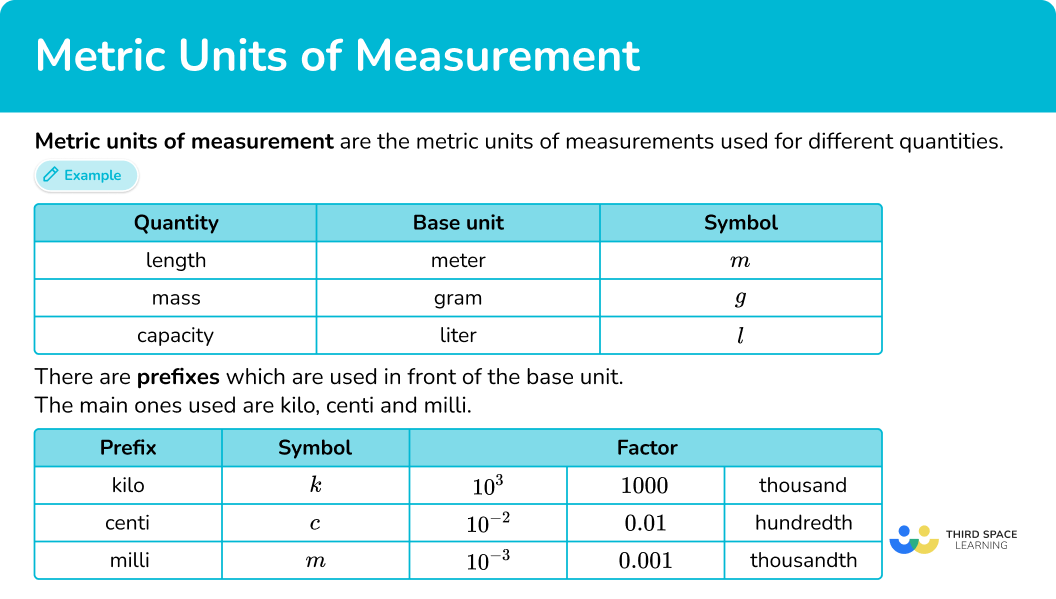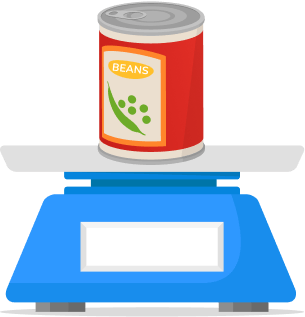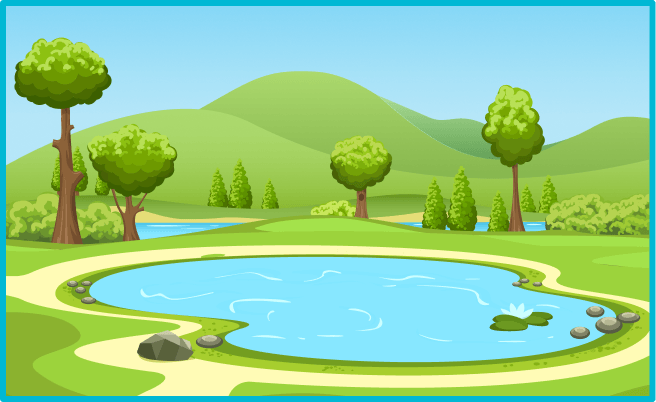# Metric units of measurement

Here you will learn about metric units of measurement. You will look at common units of measurement in the metric system of measurement and learn about their relative sizes.

Students will first learn about metric units of measurement as part of measurement and data in 4 th grade.

## What are metric units of measurement?

Metric units of measurement are the international system of units (SI units) and include the standard units of length (meter), mass (gram), capacity (liter) and time (second).

The metric system is based on powers of 10 (the decimal system). The metric prefixes along with the basic unit vocabulary help identify their standard unit and size.

The prefix “milli” means \cfrac{1}{1000} \, th.

For example,

A millimeter is \cfrac{1}{1000} \, th of a meter.

A milliliter is \cfrac{1}{1000} \, th of a liter.

The prefix “centi” means \cfrac{1}{100} \, th.

For example,

A centimeter is \cfrac{1}{100} \, th of a meter.

A centiliter is \cfrac{1}{100} \, th of a liter.

The prefix “deci,” “deca,” or “deka” means \cfrac{1}{10} \, th.

For example,

A decimeter is \cfrac{1}{10} \, th of a meter.

A decigram is \cfrac{1}{10} \, th of a gram.

The prefix “kilo” means 1,000 times larger.

For example,

A kilometer is 1,000 times larger than a meter.

A kilogram is 1,000 times larger than a gram.

### Relative sizes for metric unit measurements

Since there are many different units for measuring, it is helpful to think about each unit in relation to something in the real world. Let’s look at some common units and their relative sizes.

One way to measure an object is length – the distance from one point to another. In the metric system, a meter is the base unit for length.

One way to measure an object is mass – the weight of an object. In the metric system, a gram is the base unit for mass.

Note: A tonne is also referred to as a metric ton, but is NOT the same as a customary ton (2,000 pounds ).

One way to measure an object is capacity – how much space something takes up. In the metric system, a liter is the base unit for capacity (also referred to as the base unit of volume).

One way to measure an event is time – the length of an event with a clear starting and ending moment. In the metric system, a second is the base unit for time.

Note: All of these time measurements are very small, and it can be harder to tell the difference between them on our own.

### What are metric units of measurement?## Common Core State Standards

How does this relate to 4 th grade math?

• Grade 4 – Measurement and Data (4.MD.A.1)
Know relative sizes of measurement units within one system of units including km, m, cm; kg, g; lb, oz.; l, ml; hr, min, sec. Within a single system of measurement, express measurements in a larger unit in terms of a smaller unit. Record measurement equivalents in a two-column table.

For example, know that 1{~ft} is 12 times as long as 1 {~in}. Express the length of a 4{~ft} snake as 48{~in}. Generate a conversion table for feet and inches listing the number pairs (1, 12), (2, 24), (3, 36), …

## How to choose a metric unit of measurement

In order to choose a metric unit of measurement:

1. Decide if the measurement is length, mass, capacity or time.
2. Consider the size.
3. Write down the most reasonable choice.

## Metric units of measurements examples

### Example 1: units of length

What are the most appropriate units to measure the width of a hand-held calculator?

1. Decide if the measurement is length, mass, capacity or time.

Width is a measurement of length, so you need to consider the measurement units based on the meter (m).

2Consider the size.

Thinking about the size of a hand-held calculator, a meter (about the length of a baseball bat) is too large, or a unit and millimeters (about the width of the tip of a pencil) are too small.

3Write down the most reasonable choice.

Centimeters (each about the width of a fingernail) is the most appropriate unit to measure the width of a hand-held calculator.

### Example 2: units of length

What are the most appropriate units to measure the distance between London and Paris?

Distance is a measurement of length, so you need to consider the measurement units based on the meter (m).

The distance from these two cities, which are not in the same country, is much greater than a meter (m).

Kilometers (km) is the most appropriate unit to measure the distance between London and Paris.

### Example 3: units of mass

What are the most appropriate units to measure the weight of a large dog?

Weight is a measurement of mass, so you need to consider the measurement units based on the gram (g).

A tonne (about the weight of a small car) is too big. A gram (about the weight of a penny) is too small.

A kilogram (each about the weight of a pineapple) is the most appropriate unit of measurement for the weight of a large dog.

### Example 4: units of mass

What are the most appropriate units to measure the weight of a pencil?

Weight is a measurement of mass, so you need to consider the measurement units based on the gram (g).

A kilogram (about the weight of a cantaloupe) is too big.

A gram (each about the weight of a paper clip) is the most appropriate unit of measurement for the mass of a pencil.

### Example 5: units of capacity (volume)

What are the most appropriate units to measure the amount of tea in a tea cup?

The amount of tea is a measurement of capacity, so you need to consider the measurement units based on the liter (l).

A liter (about the amount in a quart of milk) is too large.

Milliliters (each about 1 drop of water) is the most appropriate unit of measurement for the capacity of a tea cup.

### Example 6: units of capacity (volume)

What are the most appropriate units to measure the amount of water in a pond?

The amount of water is a measurement of capacity, so you need to consider the measurement units based on the liter (l).

A milliliter (about 1 drop of water) is too small.

Liters (each about the amount of 4 cans of soda) is the most appropriate unit of measurement for the capacity of a pond.

### Teaching tips for metric units of measurement

• Worksheets can be helpful for this skill, but not before students have real life experiences with the different metric units. It is much easier to understand metric units if their use is connected to a memory or experience.

• Putting up a “units of the metric system” poster in the classroom can be helpful for students to reference as they are learning the different types of measurements. Particularly if they are used to using the customary system and have less exposure to the metric system.

• This skill should be taught before metric conversions (unit conversions). Once students have experience using metric units and understand their relative size, they are ready to start converting units.

### Easy mistakes to make

• Confusing metric and customary units
A common error is to confuse metric units with customary units of measurement. There are conversion rates between them, but they are different units in different systems.
For example,
1{~inch} ≈ 2.5{~cm}
1{~foot} ≈ 30{~cm}
1{~mile} ≈ 1.5{~km}

• Thinking the amount of substance changes when a measurement is converted
When a measurement is converted to another unit, the number representing it becomes smaller or larger. This is not because the amount being measured changed, but the way it was being measured changed.
For example,
\$1 = 20 nickels Converting \$1 to 20 nickels, increases the 1 to a 20, but since nickels are a smaller unit the overall value of \$1 has not changed. The conversion just shows that it takes 20 nickels to equal \$1.

• Thinking the millionth is the smallest unit in the metric system
While this unit is very, very small, there are other units even smaller. Because the conversion factors in the metric system are based on the decimal system, and the decimal system goes on forever (decreasing by powers of 10 infinitely), in theory there is no end to the units that can be measured in the metric system.
The metrics units that currently exist are based off the tools we have available to measure – smaller and smaller units often need more sophisticated measuring tools.

• Forgetting about less common units
There are units that are commonly used in daily life, like liter and milliliter. But there are also units that exist between (or above and below) these commonly used units. They are easy to forget since they are not used often.
For example,
Between liter and milliliter are units between deciliter and centiliter.

Deciliters are 10 times smaller than liters. And centiliters are 10 times smaller than deciliters.

### Practice metric units of measurement questions

1) Choose the most reasonable metric unit to measure:

The height of a buildingmeterskilogramsinchesmillimetersYou need a unit for length – so kilograms can be ruled out.

You need a metric unit – so inches can be ruled out.

A building is large, and a millimeter is about the thickness of 10 sheets of paper, so it is too small.

A meter is about the length of a guitar. Meters is the most reasonable unit to measure the height of a building.

2) Choose the most reasonable metric unit to measure:

The weight of a can of beanskilogramsouncesmillilitersgramsYou need a unit for mass – so milliliters can be ruled out.

You need a metric unit – so ounces can be ruled out.

A kilogram is about the weight of 3 chapter books, so it is too large.

A gram is about the weight of a penny. Grams is the most reasonable unit to measure the weight of a can of beans.

3) Choose the most reasonable metric unit to measure:

The thickness of a chapter bookmetersmillimetersyardsmillilitersYou need a unit for length – so milliliters can be ruled out.

You need a metric unit – so yards can be ruled out.

A meter is about the length of a yard stick, so it is too large.

A millimeter is about the thickness of an ID card. Millimeters is the most reasonable unit to measure the thickness of a chapter book.

4) Choose the most reasonable metric unit to measure:

The volume of a medicine dropperkilogramspintsmilliliterslitersYou need a unit for capacity (volume) – so kilograms can be ruled out.

You need a metric unit – so pints can be ruled out.

A liter is about the amount in a large soda bottle, so it is too large.

A milliliter is about the amount in a drop of water. Millimeters is the most reasonable unit to measure the volume of a medicine dropper.

5) Choose the most reasonable metric unit to measure:

The weight of a sheepgramskilogramspoundscentimetersYou need a unit for mass – so centimeters can be ruled out.

You need a metric unit – so pounds can be ruled out.

A gram is about the weight of a feather, so that is too small.

A kilogram is about the weight of a bunch of bananas. Kilograms is the most reasonable unit to measure the weight of a sheep.

6) Choose the most reasonable metric unit to measure:

The volume of a lakekilometerslitersmillilitersgallonsYou need a unit for capacity – so kilometers can be ruled out.

You need a metric unit – so gallons can be ruled out.

A milliliter is about the amount in a drop of water.

A liter is about the amount of shampoo in a full bottle. Liter is the most reasonable unit to measure the volume of a lake.

## Metric units of measurement FAQs

Can you convert between the metric system and the customary system?

Yes, there are defined conversions that allow you to move between the two.

What are other basic units of measurement?

Besides the units mentioned on this page, there are additional basic units in the International System of Units. Electric currents are measured by amperes. Thermodynamic temperature is measured in kelvin. Luminous intensity is measured in candela. And moles are used to measure the amount of substance.

## Still stuck?

At Third Space Learning, we specialize in helping teachers and school leaders to provide personalized math support for more of their students through high-quality, online one-on-one math tutoring delivered by subject experts.

Each week, our tutors support thousands of students who are at risk of not meeting their grade-level expectations, and help accelerate their progress and boost their confidence.